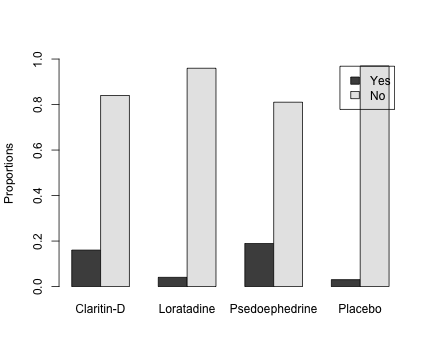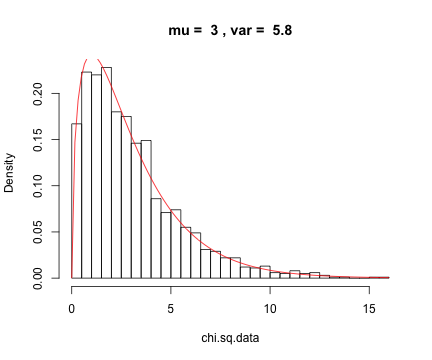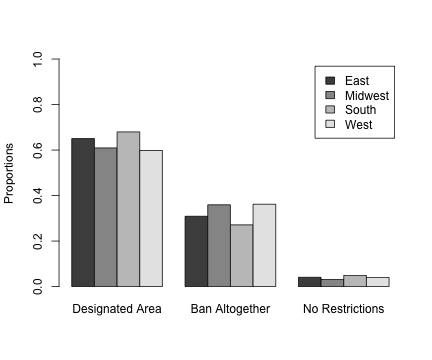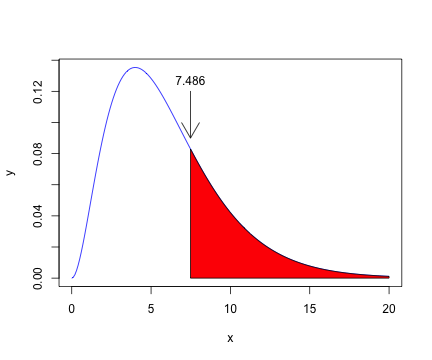# Chi-Square Test of Homogeneity

In this activity we will introduce the Chi-Square Test of Homogeneity. We begin by sharing some data from Aliaga in Example 14.3, which compares some of the adverse effects of drugs assigned for seasonal allergy relief. In this particular experiment, there were four different populations, one used Claritin-D, a second used Loratadine, a third used Pseudoephedrine, and a fourth was assigned a Placebo. Note that these are the column names in the data table containing the observed values.

$\begin{array}{ccccc} & \text{Claritin-D} & \text{Loratadine} & \text{Pseudoephedrine} & \text{Placebo}\\\hline \text{Yes} & 164 & 22 & 104 & 28\\ \text{No} & 859 & 521 & 444 & 894 \end{array}$

The members of each column group were asked if they experienced isomnia. The first row is a qualitative or categorical variable containing the number of patients who did experience insomnia and the second row contains the number of patients who did not experience insomnia.

The word “homogeneous” means “of the same kind or nature,” or “essentially alike.” Thus, the test of homogeneity is asking whether the distributions of yes and no for each population is the same. Hence, our null and alternative hypotheses can be stated as follows.

• $$H_0$$: The distribution for isomnia experience is the same for each treatment group.

• $$H_1$$: The distribution for isomnia experience is not the same for each treatment group.

We begin by entering the data in R is a matrix. Recall that, by default, R fills a matrix by column, so enter the first column, then the second column, then the third column, and finally the fourth column.

Observed=matrix(c(164,859,22,521,104,444,28,894),nrow=2)
Observed

##      [,1] [,2] [,3] [,4]
## [1,]  164   22  104   28
## [2,]  859  521  444  894


We can add row and column names.

colnames(Observed)=c("Claritin-D","Loratadine","Psedoephedrine","Placebo")
rownames(Observed)=c("Yes","No")
Observed

##     Claritin-D Loratadine Psedoephedrine Placebo
## Yes        164         22            104      28
## No         859        521            444     894


Let's add a barplot to investigate the data. Recall (see: Displaying Relationships between Two Qualitative Variables) that R's prop.table command will either divide each entry in the matrix by its corresponding row sum or corresponding column sum. The command prob.tabl(Observed,1) would divide each entry in a particular row by the sum of the elements in that row. What we want to do is divide each entry in a particular column by the sum of the entries in that column, which gives us the distribution of “Yes” and “No” for that particular column.

prop.table(Observed,2)

##     Claritin-D Loratadine Psedoephedrine Placebo
## Yes     0.1603    0.04052         0.1898 0.03037
## No      0.8397    0.95948         0.8102 0.96963


Thus, we see that about 16% of those taking Claritin-D experienced insomnia, while approximately 84\% did not. Secondly, approximately 4% of those taking Loratadine experience insomnia, while 96% did not. Let's create a barplot with this proportions.

barplot(prop.table(Observed,2),beside=TRUE,legend.text=TRUE,
ylim=c(0,1),ylab="Proportions")Note that the distributions in each column seem different, which makes us think the null hypothesis should be rejected. However, we need to first see if the data is significant with the Chi-Square test before we make a decision.

## Expected Counts

We can ask R to sum the rows and columns (as well as the total pieces of data) with R's addmargins command.

x=addmargins(Observed)
x

##     Claritin-D Loratadine Psedoephedrine Placebo  Sum
## Yes        164         22            104      28  318
## No         859        521            444     894 2718
## Sum       1023        543            548     922 3036


Note that there is a total of $$n=3036$$ patients, 318 of which answered “Yes, I have experienced isomina.” this means that the percentage of total patients that have experienced insomnia is calculated as follows:

p.yes=318/3036
p.yes

##  0.1047


Because 2718 of 3036 patients answered “No, I have not experienced insomnia”, the percentage of total patients that have not experienced insomnia is calculated as follows:

p.no=2718/3036
p.no

##  0.8953


To perform the test and calculate a $$p$$-value, we assume that the null hypothesis is true. That is, the distributions of each population are the same, which means 10.47% of each population experienced isomnia and 89.53% of each population did not experience insomnia. For example, there are 1023 patients taking Claritin-D, so the expected number of these patients who experienced insomnia and did not experience insomnia is calculated as follows:

Claritin.D=1023*c(p.yes,p.no)
Claritin.D

##  107.2 915.8


Because there were 543 patients taking Loratadine, 548 patients taking Pseudoephedrine, and 922 patients taking Placebo, we can calculate the distributions of Yes and No for each of these groups in a similar manner.

Loratadine=543*c(p.yes,p.no)
Pseudoephedrine=548*c(p.yes,p.no)
Placebo=922*c(p.yes,p.no)


We can now create a matrix by combining each of these vectors with R's cbind command. We can also add row names.

Expected=cbind(Claritin.D,Loratadine,Pseudoephedrine,Placebo)
rownames(Expected)=c("Yes","No")
Expected

##     Claritin.D Loratadine Pseudoephedrine Placebo
## Yes      107.2      56.88            57.4   96.57
## No       915.8     486.12           490.6  825.43


Now that we have both the observed and expected counts, we can calculate the test statistic.

## Calculating the Test Statistic

The Chi-Square test statistic is calculated in a manner similar to the way it was calculated in the Chi-Square test for Goodness of Fit. That is:

$\chi_{\text{OBS}}^2=\sum_{\text{all cells}}\frac{(O-E)^2}{E}$

That is, we need to compute the following sum: \begin{align*} \chi_{\text{OBS}}^2 &=\frac{(164-107.2)^2}{107.2} +\frac{(22-56.88)^2}{56.88} +\frac{(104-57.4)^2}{57.4} +\frac{(28-96.57)^2}{96.57}\\ &\hspace{2cm}+\frac{(859-915.8)^2}{915.8} +\frac{(521-486.12)^2}{486.12} +\frac{(444-490.6)^2}{490.6} +\frac{(894-825.43)^2}{825.43}\\ &\approx154.2 \end{align*}

We can calculate the test statistic much quicker using code similar to that used in the Goodness of Fit test.

chi.sq=sum((Observed-Expected)^2/Expected)
chi.sq

##  154.2


## The Chi-Square Distribution

Aliaga again claims that if we were to were to repeat the sampling process many times, each time computing the $$\chi^2$$ test statistic, storing the test statistics, then a histogram of the results should be a Chi-Square distribution. Furthermore, the degree of freedom of the distribution is calculated with the formula $df=(R-1)(C-1),$ where $$R$$ is the number of rows and $$C$$ is the number of columns. In this case, we have 2 rows and 4 columns, so the degrees of freedom should be: $df=(2-1)(4-1)=3$ One last Aliaga claim is the fact that the mean of the Chi-Square distribution is equal to the degrees of freedom and the variation is equal to twice the degrees of freedom. Thus, in this case, the mean and variation are: $\mu=3\qquad\text{and}\qquad\sigma^2=6$ So, we wrote a script to test whether this claim is accurate. Here is the result.

p=0.10
q=1-p

chi.sq.data=numeric()

M=2000
samp.size=1000

for (i in 1:M) {
observed=matrix(rep(0,8),nrow=2)
for (j in 1:4) {
data = sample(c("Yes","No"),
size=samp.size,
replace=TRUE,
prob=c(p,q))
observed[1,j]=sum(data=="Yes")
observed[2,j]=sum(data=="No")
}
n=sum(observed)

expected=matrix(rep(0,8),nrow=2)
for (r in 1:2) {
for (c in 1:4) {
expected[r,c]=sum(observed[r,])*sum(observed[,c])/n
}
}

chi.sq.data[i]=sum((observed-expected)^2/expected)

}

mu=round(mean(chi.sq.data),1)
sigma.sq=round(var(chi.sq.data),1)

hist(chi.sq.data,prob=TRUE,breaks="FD",
main=paste("mu = ", mu, ", var = ", sigma.sq))Yes! The claim appears to be true.

## Calculating the $$p$$-value

We can now calculate the $$p$$-value for the test. Again, note that the direction of extreme will again be to the right.

\begin{align*} p\text{-value}&=P(\text{Observed value or more extreme under H_0})\\ &=P(\chi^2\ge154.2) \end{align*}

Note that 154.2 is completely off the plot, more than $$154.2/6=25.7$$ standard deviations from the mean. Hence, the $$p$$-value is essentially zero, but we can still compute it using the pchisq(x,df) command. Again, the degrees of freedom are calculated with $$df=(R-1)(C-1)$$. Remember, the pchisq(x,df) computes the area to the left, but the direction of extreme is to the right, so we adjust our answer by subtracting from 1.

R=2
C=4
df=(R-1)*(C-1)
1-pchisq(chi.sq,df)

##  0


## Using R's chisq.test

Now, let's test our results using R's chisq.test.

res=chisq.test(Observed)
res

##
##  Pearson's Chi-squared test
##
## data:  Observed
## X-squared = 154.2, df = 3, p-value < 2.2e-16


Note that these numbers are the same as the numbers computed above. There are also a serious number of results stored in the variable res. Here is how you can obtain of list of what is present.

summary(res)

##           Length Class  Mode
## statistic 1      -none- numeric
## parameter 1      -none- numeric
## p.value   1      -none- numeric
## method    1      -none- character
## data.name 1      -none- character
## observed  8      -none- numeric
## expected  8      -none- numeric
## residuals 8      -none- numeric
## stdres    8      -none- numeric


Are the expected values stored in res?

res\$expected

##     Claritin-D Loratadine Psedoephedrine Placebo
## Yes      107.2      56.88           57.4   96.57
## No       915.8     486.12          490.6  825.43


My goodness, that would have saved us a bit of time!

# A Second Example

This example was taken from an excellent text called Statistics in Action by Ann Watkins.

Th Gallup Poll Monthly reported on a survey built around the question “What is your opinion regarding smoking in public places?” On the workplace part of the survey, respondents were asked to make on of three choices as to the policy they favored on smoking in the workplace. The percentages of the sample responding to these three choices across four geographic regions are shown in the following table. $\begin{array}{ccccc} & \textbf{Designated Area} & \textbf{Ban Altogether} & \textbf{No Restrictions} & \textbf{Sample Size}\\ \textbf{East} & 65\% & 31\% & 4\% & 246\\ \textbf{Midwest} & 61\% & 36\% & 3\% & 256\\ \textbf{South} & 68\% & 27\% & 5\% & 306\\ \textbf{West} & 60\% & 36\% & 4\% & 199\\ \end{array}$

First, note that the cell data are given as percents. Our first step is to find the observed number of counts for each cell. The first row is the survey in the east, which has a sample of size 246. Thus, we can compute the counts for Designated Area, Ban Altogether, and No Restrictions, by applying each given percent to the sample size, rounding to the nearest unit.

East=round(c(.65,.31,.04)*246,0)
East

##  160  76  10


Now, the same strategy for the remaining regions.

Midwest=round(c(.61,.36,.03)*256,0)
South=round(c(.68,.27,.05)*306,0)
West=round(c(.60,.36,.04)*199,0)


Now we can use R's rbind command to bind these in a matrix by row.

Observed=rbind(East,Midwest,South,West)
Observed

##         [,1] [,2] [,3]
## East     160   76   10
## Midwest  156   92    8
## South    208   83   15
## West     119   72    8


colnames(Observed)=c("Designated Area","Ban Altogether","No Restrictions")
Observed

##         Designated Area Ban Altogether No Restrictions
## East                160             76              10
## Midwest             156             92               8
## South               208             83              15
## West                119             72               8


Now, let's state the null and alternative hypotheses.

• $$H_0$$: The distribution of opinion is the same for each region.
• $$H_1$$: The distribution of opinion is \textbf{not} the same for each region.

Let's add a barplot to investigate the data. This time our population categories are placed in each row, East, Midwest, South, and West, but the categorical responses are placed in each column. Thus, what we want to view is the distribution of each row, so this time we will use the command prop.table(Observes,1) to divide each entry in a particular row by the sum of the entries in that row.

prop.table(Observed,1)

##         Designated Area Ban Altogether No Restrictions
## East             0.6504         0.3089         0.04065
## Midwest          0.6094         0.3594         0.03125
## South            0.6797         0.2712         0.04902
## West             0.5980         0.3618         0.04020


Thus, approximately 65% of the people from the East region prefer a Designated Area, approximately 31% prefer a Ban Altogether, and approximately 4% prefer No Restrictions. From the Midwest region, approximately 61% of the people from the East region prefer a Designated Area, approximately 36% prefer a Ban Altogether, and approximately 3% prefer No Restrictions. Let's create a barplot with these proportions.

barplot(prop.table(Observed,1),beside=TRUE,legend.text=TRUE,
ylim=c(0,1),ylab="Proportions")The distributions of opinion for Designated Area, Ban Altogether, and No Restrictions seem to be rather similar for East, Midwest, South, and West, making us think we'll fail to reject the null hypothesis. However, we need to run the Chi-Square test for homogeneity to compute the $$p$$-value and make a decision at the $$\alpha=0.05$$ level.

## Expected Counts

First, use R's addmargins command to provide sums for each row and column of the Observed data.

x=addmargins(Observed)
x

##         Designated Area Ban Altogether No Restrictions  Sum
## East                160             76              10  246
## Midwest             156             92               8  256
## South               208             83              15  306
## West                119             72               8  199
## Sum                 643            323              41 1007


Note that there are a total of 1007 people surveyed, 643 of which prefere a Designated Area, 323 of which prefer Ban Altogether, and 41 of which prefer No Restrictions. We will store these proprotions in p.DA, p.BA, and p.NR.

p.DA=643/1007
p.BA=323/1007
p.NR=41/1007


Now, there are a total of 246 people from the east surveyed, so the expected counts for each category will be found by multiply 246 by p.DA, p.BA, and p.NR, rounding to the nearest unit.

East=246*c(p.DA,p.BA,p.NR)
East

##  157.08  78.91  10.02


There are 256, 306, and 199 people surveyed from the Midwest, South, and West. We won't round our answers this time in the hopes that our calculations will match the chisq.test at the end of this activity.

Midwest=256*c(p.DA,p.BA,p.NR)
South=306*c(p.DA,p.BA,p.NR)
West=199*c(p.DA,p.BA,p.NR)


We can now bind these by row using R's rbind command, and we can add column names.

Expected=rbind(East,Midwest,South,West)
colnames(Expected)=c("Designated Area","Ban Altogether","No Restrictions")
Expected

##         Designated Area Ban Altogether No Restrictions
## East              157.1          78.91          10.016
## Midwest           163.5          82.11          10.423
## South             195.4          98.15          12.459
## West              127.1          63.83           8.102


Now we can use $\chi_{\text{OBS}}^2=\sum_{\text{all cells}}\frac{(O-E)^2}{E}$ to compute the observed statistic.

chi.sq=sum((Observed-Expected)^2/Expected)
chi.sq

##  7.486


We can now determine the $$p$$-value. Remember, the direction of extreme is to the right.

\begin{align*} p\text{-value}&=P(\text{Observed value or more extreme under H_0.})\\ &=P(\chi^2\ge7.4859) \end{align*}

Next, sketch the distribution and shade the region representing the $$p$$-value. Because we have four rows and three columns, the degrees of freedom are $$(R-1)(C-1)=(4-1)(3-1)=6$$.

x=seq(0,20,length=200)
y=dchisq(x,6)
plot(x,y,type="l",col="blue")
xx=seq(7.486,20,length=100)
yy=dchisq(xx,6)
polygon(c(7.486,xx,20),c(0,yy,0),col="red")
arrows(7.486,0.12,7.486,0.09)
text(7.486,0.12,"7.486",pos=3)We can now use the pchisq(x,df) command to compute the $$p$$-value. Remember, this command computes the area to the left, so we make the usual adjustment by subtracting the result from 1.

R=4
C=3
df=(R-1)*(C-1)
1-pchisq(chi.sq,df)

##  0.2782


## Checking Our Work with R's chisq.test

Now, let's check our results with R's chisq.test.

chisq.test(Observed)

##
##  Pearson's Chi-squared test
##
## data:  Observed
## X-squared = 7.486, df = 6, p-value = 0.2782


Note that the $$\chi^2=7.486$$ and the $$p$$-value equals 0.2782, which are identical found with our calculations above.

## Decision

At the $$\alpha=0.05$$ level of significance, because our $$p$$-value is not less than $$\alpha=0.05$$, we choose to not reject the null hypothesis.